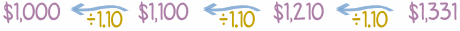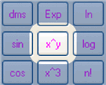# Present Value (PV)

Money now is more valuable than money later on.

Why? Because you can use money to make more money!

You could run a business, or buy something now and sell it later for more, or simply put the money in the bank to earn interest.

### Example: You can get 10% interest on your money.

So \$1,000 now can earn \$1,000 x 10% = \$100 in a year.

Your \$1,000 now can become \$1,100 in a year's time.

## Present ValueSo \$1,000 now is the same as \$1,100 next year (at 10% interest).We say the Present Value of \$1,100 next year is \$1,000

Because we could turn \$1,000 into \$1,100 (if we could earn 10% interest).

Now let us extend this idea further into the future ...

## How to Calculate Future Payments

Let us stay with 10% Interest. That means that money grows by 10% every year, like this:So:

• \$1,100 next year is the same as \$1,000 now.
• And \$1,210 in 2 years is the same as \$1,000 now.
• etc

In fact all those amounts are the same (considering when they occur and the 10% interest).

## Easier Calculation

But instead of "adding 10%" to each year it is easier to multiply by 1.10 (explained at Compound Interest):So we get this (same result as above):## Future Back to Now

And to see what money in the future is worth now, go backwards (dividing by 1.10 each year instead of multiplying):### Example: Sam promises you \$500 next year, what is the Present Value?

To take a future payment backwards one year divide by 1.10

So \$500 next year is \$500 ÷ 1.10 = \$454.55 now (to nearest cent).

The Present Value is \$454.55

### Example: Alex promises you \$900 in 3 years, what is the Present Value?

To take a future payment backwards three years divide by 1.10 three times

So \$900 in 3 years is:

\$900 ÷ 1.10 ÷ 1.10 ÷ 1.10
\$900 ÷ (1.10 × 1.10 × 1.10)
\$900 ÷ 1.331
\$676.18 now (to nearest cent).

## Better With Exponents

But instead of \$900 ÷ (1.10 × 1.10 × 1.10) it is better to use exponents (the exponent says how many times to use the number in a multiplication).

### Example: (continued)

The Present Value of \$900 in 3 years (in one go):

\$900 ÷ 1.103 = \$676.18 now (to nearest cent).

As a formula it is:

PV = FV / (1+r)n

• PV is Present Value
• FV is Future Value
• r is the interest rate (as a decimal, so 0.10, not 10%)
• n is the number of years

### Example: (continued)

Use the formula to calculate Present Value of \$900 in 3 years:

PV = FV / (1+r)n
PV = \$900 / (1 + 0.10)3 = \$900 / 1.103 = \$676.18 (to nearest cent).Exponents are easier to use, particularly with a calculator. For example 1.106 is quicker than 1.10 × 1.10 × 1.10 × 1.10 × 1.10 × 1.10

Let us use the formula a little more:

### Example: What is \$570 next year worth now, at an interest rate of 10% ?

PV = \$570 / (1+0.10)1 = \$570 / 1.10 = \$518.18 (to nearest cent)

But your choice of interest rate can change things!

### Example: What is \$570 next year worth now, at an interest rate of 15% ?

PV = \$570 / (1+0.15)1 = \$570 / 1.15 = \$495.65 (to nearest cent)

Or what if you don't get the money for 3 years

### Example: What is \$570 in 3 years time worth now, at an interest rate of 10% ?

PV = \$570 / (1+0.10)3 = \$570 / 1.331 = \$428.25 (to nearest cent)

One last example:

### Example: You are promised \$800 in 10 years time. What is its Present Value at an interest rate of 6% ?

PV = \$800 / (1+0.06)10 = \$800 / 1.7908... = \$446.72 (to nearest cent)